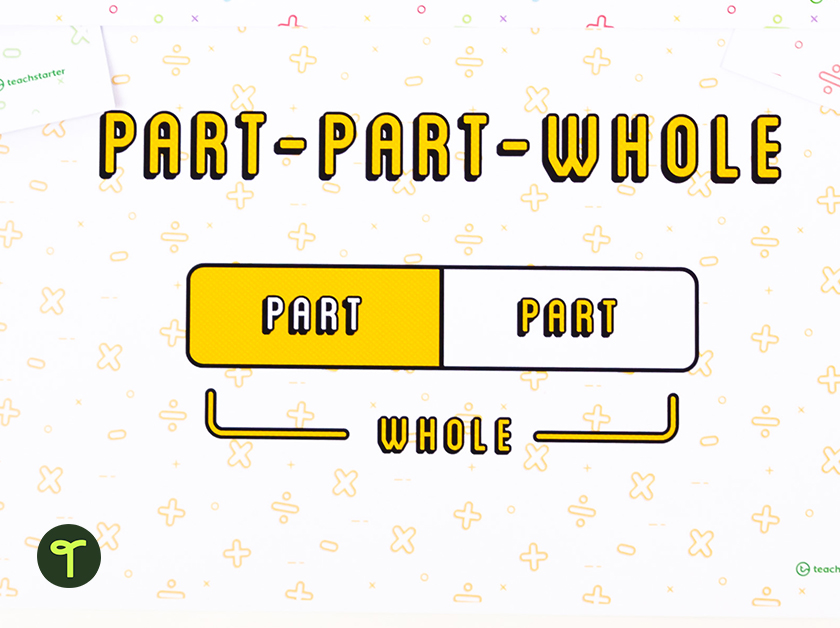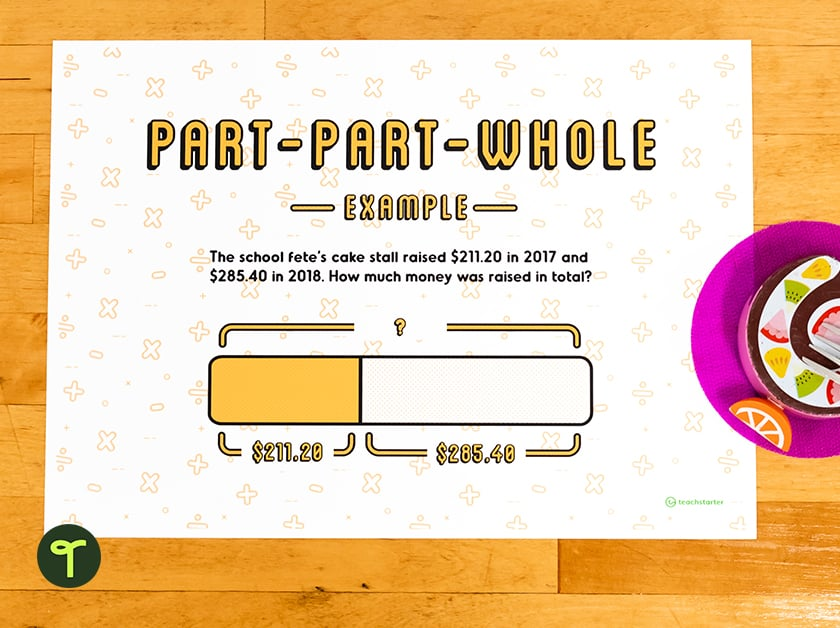Get more inspiration delivered to your inbox!

Teaching Ideas

# What Is a Bar Model? How to Use This Math Problem-Solving Method in Your Classroom

Teaching mathematical problem-solving to your students is a crucial element of mathematical understanding. They must be able to decipher word problems and recognize the correct operation to use in order to solve the problem correctly (rather than freaking out as soon as they see a mathematical problem in words!!!). But what is the best strategy for embedding this knowledge into your students? Enter the Bar Model Method, also known in education as a tape diagram, strip diagram, or tape model.

The model has gained traction in classrooms around the world, so you may be thinking it’s time to introduce it in your classroom. But what is the bar model all about, and what are the advantages of using this method with elementary students? The math teachers on the Teach Starter team are here with a quick primer to help you decide if this is the right path for your math class!

Explore the latest math teaching resources from Teach Starter!

## What Is a Bar Model?

The Bar Model is a mathematical diagram that is used to represent and solve problems involving quantities and their relationships to one another.

It was developed in Singapore in the 1980s when data showed Singapore’s elementary school students were lagging behind their peers in math. An analysis of testing data at the time showed less than half of Singapore’s students in grades 2-4 could solve word problems that were presented without keywords such as “altogether” or “left.” Something had to be done, and that something was the introduction of the bar model, which has been widely credited with rocketing the kids of Singapore to the top of math scores for kids all around the globe.

At its core, the bar model is an explicit teaching and learning strategy for problem-solving. The actual bar model consists of a set of bars or rectangles that represent the quantities in the problem, and the operations are represented by the lengths and arrangements of the bars. Among its strengths is the fact that it can be applied to all operations, including multiplication and division. They’re also useful when it comes to teaching students more advanced math concepts, such as ratios and proportionality.

The Bar Model combines the concrete (drawings) and the abstract (algorithms or equations) to help the student solve the problem.

## How to Use a Bar Model in Math

Whether you’re calling it a bar model, a strip diagram, or a tape diagram, the concept is the same – you have rectangular bars (or strips) that are laid out horizontally to represent quantities and the relationships between them.

• The bars themselves — horizontal rectangles—  represent the problem.
• The length of the bar(s) represents the quantity.
• The locations of the bars show the relationship between the quantities.

Visualizing this relationship helps students decide which operation to use to solve the problem. The student then labels the known quantities with numbers and labels the unknown quantities with question marks.The three basic structures are:

• Part-Part-Whole
• Comparison
• Equal Parts

Our printable Bar Model Poster Pack is a perfect way to introduce your students to the basics of the Bar Model!

Many teachers (particularly in the elementary grades) will recognize elements of the Bar Model as being similar to the Part-Part-Whole method we’ve been teaching forever.

The Bar Model could be looked at as an extension of this concept. It can be used by students right through elementary school (and beyond) not only to solve addition and subtraction problems but to tackle multiplication and division word-based problems as well.## Teaching with the Bar Model

The Bar Model can easily be incorporated into your elementary math instruction, from simple addition to more complex multiplication and division and so on. Here are just a few ideas from our teacher team:

• Use part–whole bar models to show word problems with a missing number element to teach addition or subtraction.
• Incorporate into your CUBES strategy — The E in CUBES stands for Evaluate and Draw. As a Bar Model is a drawing, this is the perfect place to use it.
• Use the bar model method to help students see how a bar must be cut into equal parts when multiplying and dividing.
• Help students visualize fractions with the bar model — Use the rectangles to help students see how the fractions relate to whole numbers by showing the relationship between the numerator and denominator.

#### Explore our complete collection of curriculum-aligned resources for teaching about operations!

Banner image via Shutterstock/Komuso Colorsandia